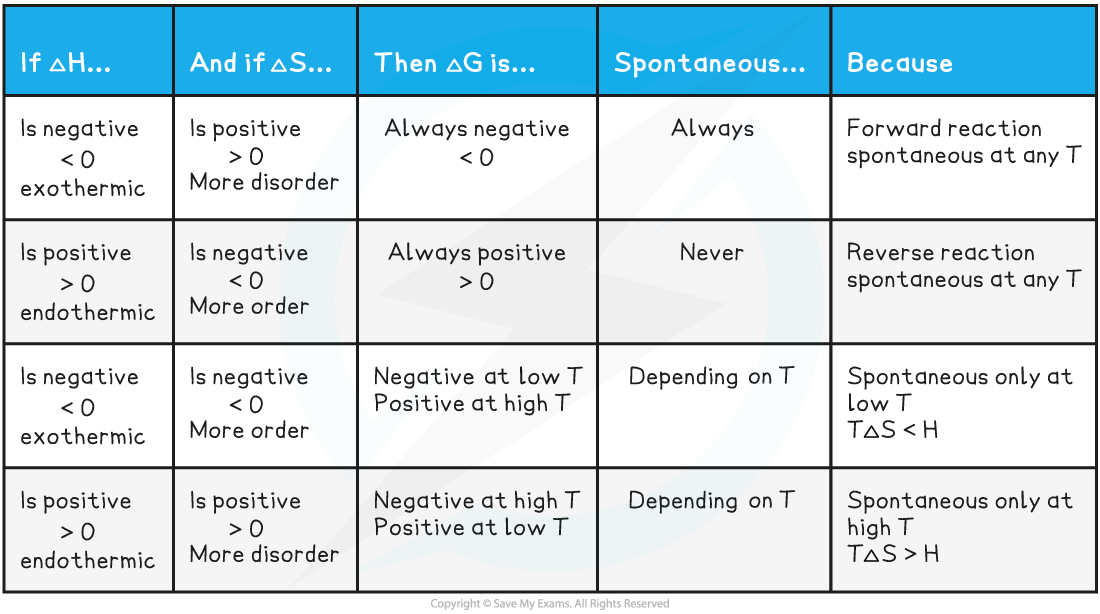# Edexcel A Level Chemistry:复习笔记5.4.3 Gibbs Free Energy

### Feasibility of Reactions

#### Gibbs free energy

• The feasibility of a reaction is determined by two factors
• The enthalpy and entropy change
• The two factors come together in a fundamental thermodynamic concept called the Gibbs free energy (G)
• The Gibbs equation is:

ΔGꝋ = ΔHreactionꝋ – TΔSsystemꝋ

• The units of ΔGꝋ are in kJ mol–1
• The units of ΔHreactionꝋare in kJ mol–1
• The units of T are in K
• The units of ΔSsystemꝋ are in J K-1 mol–1(and must therefore be converted to kJ K–1 mol–1by dividing by 1000)

• For a reaction to be feasible, ΔGꝋ must be equal or less than zero

### Gibbs Free Energy Calculations

#### Calculating ΔGΘ

• This can be done by using the Gibbs equation, using enthalpy change, ΔHꝋ, and entropy change, ΔSꝋ, values

#### Worked Example

ΔGꝋfrom ΔHꝋand ΔSꝋvalues

Calculate the free energy change for the following reaction:

2NaHCO3 (s) → Na2CO3 (s) + H2O (l) + CO2 (g)

• ΔHꝋ = +135 kJ mol-1
• ΔSꝋ = +344 J K-1 mol-1

Step 1: Convert the entropy value in kilojoules

ΔSꝋ = +344 J K-1 mol-1  ÷ 1000 = +0.344 kJ K-1 mol-1

Step 2: Substitute the terms into the Gibbs Equation

ΔGꝋ= ΔHreactionꝋ– TΔSsystemꝋ

= +135 – (298 x 0.344)

= +32.49 kJ mol-1

The temperature is 298 K since standard values are quoted in the question

• Rearranging the Gibbs equation allows you to determine the temperature at which a non-spontaneous reaction become feasible

ΔGꝋ = ΔHreactionꝋ - TΔSsystemꝋ

• Remember, for a reaction to be feasible ΔGΘꝋmust be zero or negative

0 = ΔHꝋ - TΔSꝋ

ΔHꝋ = TΔSꝋ

T = ΔHꝋ ÷ ΔSꝋ

#### Worked Example

At what temperature will the reduction of aluminium oxide with carbon become spontaneous?

Al2O3(s) + 3C(s)→ 2Al(s) + 3CO(g)

ΔHꝋ = +1336 kJ mol-1

ΔSꝋ = +581 J K-1 mol-1

• If ΔGꝋ = 0 then T = ΔHꝋ ÷ ΔSꝋ
• Covert ΔSꝋ to kJ K-1 mol-1 by dividing by 1000
• T = 1336 ÷ (581/1000)
• T = 2299 K

#### Temperature and Gibbs free energy

• When ΔGꝋ is negative, the reaction is spontaneous / feasible and likely to occur
• When ΔGꝋ is positive, the reaction is not spontaneous / feasible and unlikely to occur
• We can also look at the the values for enthalpy change, ΔH, and entropy change, ΔS
• Depending on the value for ΔH and ΔS we can determine whether the reaction is spontaneous at a given temperature (T)

Summary for temperature and Gibbs free energy